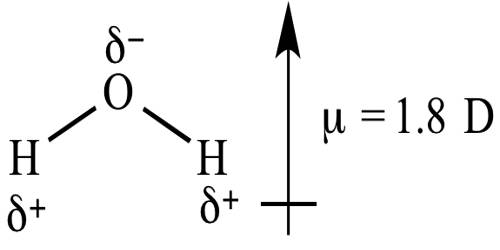# Dipole Moment and Molecular Structure

Dipole Moment and Molecular Structure

Dipole moments happen when there is a disconnection of charge. They can happen between two ions in an ionic bond or between atoms in a covalent bond; dipole moments occur from differences in electronegativity. In diatomic molecules formed by atoms of the same elements, e.g. H2, O2, Cl2 the bonding electrons forming the covalent bond are equally attracted by the two atoms joined by the bond.

If in a diatomic molecule the bonding electron pair is unequally attracted the by the two atoms, namely in HF, then there is the separation of charge in the molecule, the bonding electrons spending more time near the fluorine atom. This happens because of the difference in the electronegativity of the two atoms joined by the bond. As a result of the HF molecule, the more electronegative fluorine atom acquires partial negative charge, δ-, and the hydrogen atom acquires an equal amount of positive, δ+, charge as shown below,

δ+     δ

H   –   F

Or, more precisely it can be shown as

δ+     δ

H   :   F

Such a bond where that is a separation of charge between the two atoms is called a polar bond and the diatomic molecule is said to be a polar molecule.

The shift of electron density is represented by placing a cross arrow (⇸) above the Lewis ‘dot structure’ to indicate the direction of the shift. For HF this can be represented as-

⇸

H   :   F

As the polar molecule has two electric charges of equal magnitude of opposite sign and separated by a distance it acts, as a dipole.

The magnitude of the polarity of the bond is measured by a quantity known as dipole moment represented by µ.

The dipole moment is a vector quantity with a magnitude and direction. The direction of the vector is from positive end to the negative as indicated above. Dipole moment is defined as follows;

The dipole moment of a dipole is the product of the charge q and the distance d between the centers of the two opposite and equal charges.

In other words, μ = d x q

For a non- polar molecule q = 0, and so μ = 0. The dipole moment of a polar bond is called bond moment